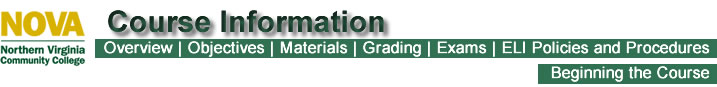BUS 221: Business Statistics- 3 Credits
Overview

In this course, you will learn descriptive statistics, a type of statistics that deals with the collection, organization, presentation and analysis of data. You will also learn concepts and theories of probability as a prelude to a course in inferential statistics that you will eventually take to make your knowledge of statistics complete. The topics covered include measures of central tendency, measures of dispersion, probability concept and distribution, sampling, statistical estimation, normal and T distribution, and hypothesis testing for means and proportion.

Top
Objectives

If you do well in this course, you will be able to:

• Collection and Presentation of Data, Frequency Distributions

• identify different methods of data collection and presentations and note advantages and disadvantages of each
• organize ungrouped data into a frequency distribution
• Measures of Central Tendency and Dispersion
• arrange ungrouped data into an array, and determine the mean, median, mode and type of skewness
• identify the modal class, median class, and class width of a given frequency distribution
• for a given data, compute the range, variance, and standard deviation
• showing formulas used and substitutions of figures into the formula
• Basic Probability Concepts
• list the elementary events and composite events of a particular business probability problem
• solve problems involving use of addition rule for both mutually exclusive and non-exclusive outcomes
• solve problems involving use of addition rule for both mutually exclusive and non-exclusive outcomes
• The Normal Distribution
• solve problems finding areas under a normal curve using a Z table
• demonstrate the use of the normal distribution in business and industrial applications such as determining cut-off scores on aptitude tests
• Discrete Probability Distributions
• state the required conditions for the use of the binomial probability distribution
• recognize the basic formula for binomial distribution applications with the use of tables, compute probabilities for appropriate applications
• recognize the conditions under which it is appropriate to use the Poisson distribution
• with the use of tables, solve problems involving the Poisson distribution
• Sampling and Sampling Distributions
• distinguish between judgment and probability samples
• recognize what is meant by simple random, systematic, stratified, and cluster samples
• define sampling distribution of the mean, and state the central limit theorem and its significance
• write the formulas for and compute the standard error of the mean and the standard error of the proportion
• Confidence, Intervals for Populations Means and Proportions, Required Sample Size
• know the difference between point estimates and interval estimates
• calculate confidence intervals for means and proportion

Top
Materials

Top

 Assignment Points Percentage of Grade Discussion Forums 30 (30 @ 1pt each) 3.8% Exercises 220 (11 @ 20pts each) 56% Quizzes 220 (11 @ 20pts each) 44% Exams 300 (3 @ 100pts each) 56% Total 770 100%

 Grading Scale A 693-770 B 616-692 C 539-615 D 462-538 F 461 and below

Top
Exams

There are three (3) exams in this course.

Top
ELI Policies and Procedures

Top
Beginning the Course

Last Updated: January 10, 2012